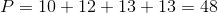## Example Questions

### Example Question #1 : Trapezoids

If a trapezoid has a height of 14, base lengths of 10 and 12, and side lengths of 13, what is its perimeter?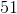Explanation:

Use the formula for perimeter of a trapezoid: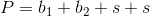Whereis the perimeter,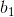and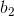are the lengths of the bases, andis the length of the side.

In this case: